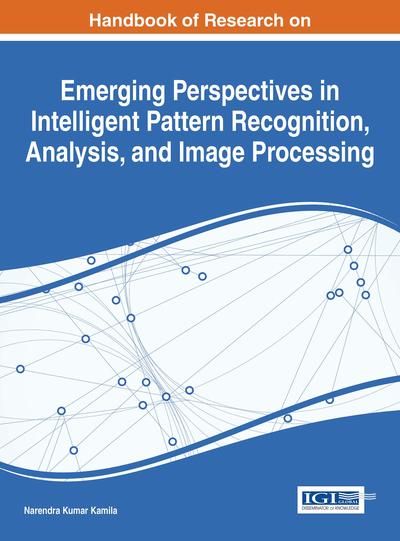# Application of Charge System Search Algorithm for Data Clustering

Yugal Kumar (Birla Institute of Technology, India) and Gadadhar Sahoo (Birla Institute of Technology Mesra, India)
DOI: 10.4018/978-1-4666-8654-0.ch018

## Abstract

This chapter presents a charged system search (CSS) optimization method for finding the optimal cluster centers for a given dataset. In CSS algorithm, while the Coulomb and Gauss laws from electrostatics are applied to initiate the local search, global search is performed using Newton second law of motion from mechanics. The efficiency and capability of the proposed algorithm is tested on seven datasets and compared with existing algorithms like K-Means, GA, PSO and ACO. From the experimental results, it is found that the proposed algorithm provides more accurate and effective results in comparison to other existing algorithms.
Chapter Preview
Top

## Introduction

Clustering is a powerful technique which is applied in finding hidden patterns and knowledge from a given set of patterns and data. It has proven its significance in many research fields such as pattern recognition (Webb, 2002; Zhou & Liu, 2008), image processing (Sonka, Hlavac, & Boyle, 2014), process monitoring (Teppola, Mujunen, & Minkkinen, 1999), machine learning (Alpaydin, 2004), quantitative structure activity relationship (Dunn III, Greenberg, & Callejas, 1976), document retrieval (Hu, Zhou, Guan, & Hu, 2008), bioinformatics (He, Pan, & Lin, 2006), image segmentation (Pappas, 1992) and many more. The aim of clustering is to group the similar items which have similar characteristics. Different similarity measures are applied to group the items into clusters but the sum of the squared distances is widely accepted similarity measure. Generally, the clustering algorithms can be classified into two groups: hierarchical clustering algorithms and partition-based clustering algorithms (Kogan, Nicholas, Teboulle, & Berkhin, 2006; Rokach, 2010). In hierarchical algorithms, a tree structure of data is formed by merging or splitting data based on similarity criterion. In partition-based algorithms, clustering is achieved by relocating data between clusters to the clustering criterion, i.e. Euclidian distance.

## Key Terms in this Chapter

Newton Law: The force acting on an object is equal to the mass of that object and its acceleration.

Charge Particles: Charged particles are the particles with an electric charge.

Clustering: It is a technique to find out hidden patterns and knowledge from a given set of patterns and data.

Gauss Law: Gauss's law is defined as the electric fields at points on the closed surface and the net charge enclosed by that surface.

Coulomb Law: Coulomb law stated that electric force between two small charged spheres (charge particles) is proportional to the inverse square of their separation distance.

## Complete Chapter List

Search this Book:
Reset